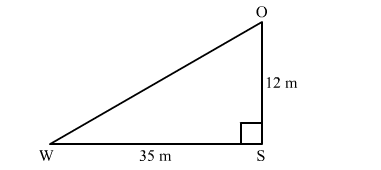# A man goes 12 m due south and then 35 m due west.Question:

A man goes 12 m due south and then 35 m due west. How far is he from the starting point?

Solution:In right triangle SOW
By using Pythagoras theorem, we have

$\mathrm{OW}^{2}=\mathrm{WS}^{2}+\mathrm{SO}^{2}$

$=35^{2}+12^{2}$

$=1225+144$

$=1369$

$\therefore \mathrm{OW}^{2}=1369$

$\Rightarrow \mathrm{OW}=37 \mathrm{~m}$

Hence, the man is 37 m away from the starting point.